#### 期刊菜单

Construction and Accuracy Analysis of Real-Time 3D Model of City-Level
DOI: 10.12677/GST.2022.104022, PDF, HTML, XML, 下载: 73  浏览: 473

Abstract: In this study, the UAV oblique photogrammetry technology is used, and the built-up area of Zhenjiang City Center and Nanshan Scenic Area are used as experimental areas, combined with Context Capture software, to analyze and process the image data of multiple flights obtained by UAV, and construct a the city-level real scene 3D model is based on the characteristic points measured by the field. In addition, the multi-granularity fusion and uniform light and color processing research were carried out on the model scene and the internal industry measures the plane and elevation values corresponding to the points with the same name in the model results, and calculates the plane ac-curacy and elevation accuracy of the statistical detection points respectively. After calculation and statistics, the error in the plane position of the model is ±0.090 meters, the error in the elevation is ±0.108 meters, which meets the accuracy requirements. The results show that the UAV oblique photogrammetry technology is feasible and accurate in the construction of city-level real 3D models, and can provide full-spatial geographic information services for the government to formulate over-all urban development strategies, urban safety operation management and other overall macro decisions.

1. 引言

2. 研究区域概况与数据源

1) 简单模型(LOD1)

2) 主体模型(LOD2)

3) 标准模型(LOD3)

4) 精细模型(LOD4)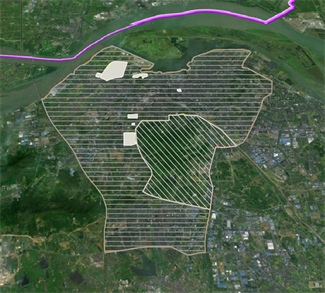Figure 1. Model construction scopeTable 1. UAV camera parameters

3. 数据处理与结果分析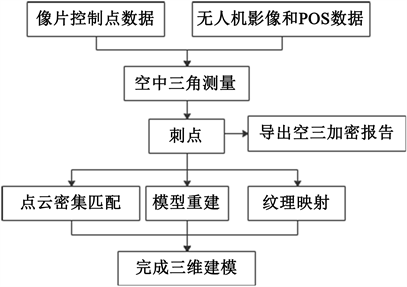Figure 2. Data processing flow chart

3.1. 三维模型构建

3.2. 多粒度三维模型融合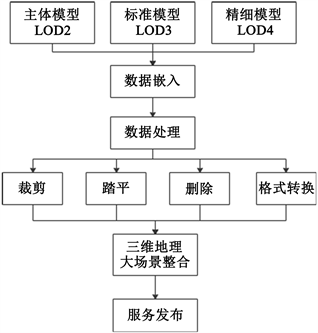Figure 3. Multi-granularity data fusion flow chart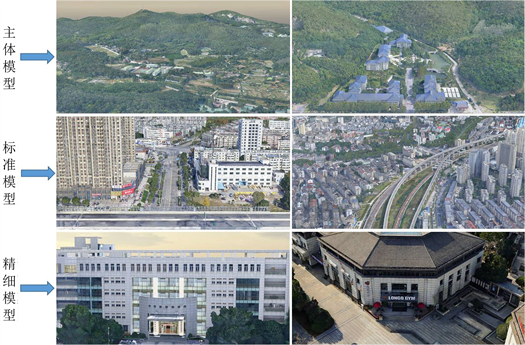Figure 4. Multi-granularity real-world 3D model in the study areaFigure 5. The effect of fusion of different granularity models

3.3. 三维模型匀光匀色处理

${D}_{j}=\underset{j=0}{\overset{n}{\sum }}\frac{{L}_{i}}{n}$ (1)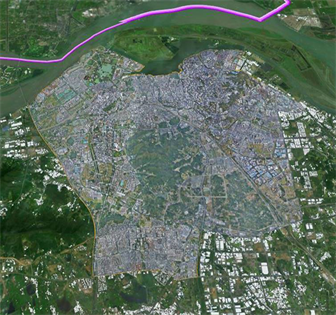Figure 6. Effect map of geographic scene after color equalization

3.4. 模型精度评价

$m=\sqrt{\frac{\sum {\left(\Delta \right)}^{2}}{n}}$ (2)Table 2. List of real 3D data detection

4. 结果与讨论

  田超, 王书阳. 基于无人机倾斜摄影测量技术的三维建模及其精度分析[J]. 资源信息与工程, 2019, 34(1): 125-126.  李星宇, 赫念学, 魏英波, 等. 复杂环境倾斜摄影与实景三维建模技术应用[J]. 测绘通报, 2020(S1): 20-24.  成李博, 李佳, 段平, 等. 顾及控制点均匀性的无人机实景三维建模精度分析[J]. 全球定位系统, 2021, 46(1): 20-27.  毕洪基, 杨荣帮. 基于低空无人机影像的城市三维建模精度评价[J]. 北京测绘, 2019, 33(5): 513-517.  刘建程, 王冠智, 金泽林, 等. 倾斜摄影测量面向城镇实景三维建模及精度分析[J]. 测绘通报, 2021(S1): 16-19.  刘丹丹, 刘江, 白阳, 等. 倾斜摄影测量三维建模及精度分析[J]. 测绘与空间地理信息, 2020, 43(7): 1-4.  周悌慧, 陈海鹏, 陈宇恒, 等. 倾斜摄影实景三维模型的质量检查分析[J]. 测绘通报, 2021(S1): 33-36.  闻彩焕, 王文栋, 王晓东, 等. 实景三维模型空间信息采集像控点布设分析[J]. 测绘通报, 2021(S1): 1-5.  李伟, 张红月, 孙衍建, 等. 地区级实景三维模型色彩均衡方法实验[J]. 山东国土资源, 2021, 37(7): 86-90.  徐思奇, 黄先锋, 张帆, 等. 倾斜摄影测量技术在大比例尺地形图测绘中的应用[J]. 测绘通报, 2018(2): 111-115.  尚浩, 宋晓帅, 李虎, 严姗姗. 济南泉域岩溶地区多源多尺度数据三维耦合模型及应用[J]. 地质学刊, 2019, 43(3): 385-392.  向泽君. 重庆全市域多源多尺度实景三维模型建设[Z]. 重庆: 重庆市勘测院, 2019-03-07.